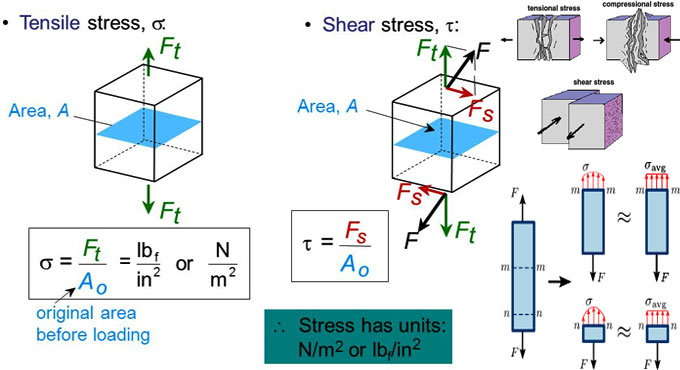# Basic variations among Shear Stress and Tensile StressStress refers to a quantity that defines how much deforming force is employed per unit area of an object. Shear and tensile stress stands for several types of stress where the forces operate on an object in a various ways.

The major variation among shear stress and tensile stress is that tensile stress is produced when a deforming force is employed at right angles to a surface, while shear stress is produced when a deforming force is employed parallel to a surface.

Definition of Tensile Stress: Tensile stress occurs in a situation when a deforming force, operating perpendicular to the surface of the object pull on the object, trying to elongate it. In this context, tensile stress belongs to a type of normal stress produced by forces perpendicular to the surface of an object.

The other type of normal stress ranges from compressive stress, where a force operates perpendicular to a surface and push in on the surface, trying to curtail it.

Suppose, the force perpendicular to the surface is taken as F and the area of the surface is A, then tensile stress (σ) is measured as follow :-

Tensile strain (ϵ) belongs to the change in length () as a fraction of the original length (x0) :

A quantity known as young modulus (E) defines how comparatively difficult it is to expand a given material. This quantity is given as follow :-

E = stress / strain = σ / ϵ
Shear stress belongs to cases where the deforming force remains parallel to a surface.

The shear stress is again identified as the ratio of the force to the area:

The variation among tensile stress and shear stress occurs in the directions of forces.

The shear strain is provided as follow :

The shear modulus is a quantity that defines how difficult it is for a material to be deformed through a shear stress. The shear modulus for a material is identified as:

Given below, common differences among Shear Stress and Tensile Stress

Direction of Forces: Forces which produce tensile stress remain at right angles to a surface. Forces which produce shear stress function parallel to a surface.

Deformation of the Object: Due to tensile stress, the objects are elongated. Due to shear stress, the one surface of an object is dislocated relating to the surface opposite to it.

Relative Strengths: Solid materials deform quickly under shear stress than under tensile stress.